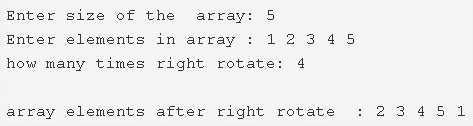# C Program To Right Rotate An Array | 4 Ways

C Program to right rotate an array – In this article, we will detail in on the various means of how to right rotate an array in C programming.

Suitable examples and sample programs have also been added so that you can understand the whole thing very clearly. The compiler has also been added with which you can execute it yourself.

• Using Standard Method
• Using Function

An array is a sequential collection of elements in a horizontal fashion. The elements in an array are defined by the user themselves.As you can see in the image uploaded above, firstly, you need to enter the size of the array.

The size of the current array mentioned is taken to be 5.

Later, enter the elements into the array.

The elements entered in this are as follows:

1 2 3 4 5

Then, mention the number of times to right rotate.

In this pic, the number is 4.

So, the final array would be like:

2 3 4 5 1

Thus, the multiple methods to do so in C programming are as follows:

## Using Standard Method

1. Read the array size and store the size into the variable n.

2) Read the entered elements and store the elements in the array a[] as scanf(“%d”,&a[i]) using for loop.

3) Read the k value which represents how many times right rotate the array.

4) Right, rotate the array up to k times as follows

for loop iterates from i=0 to i<k

a) Store the last element into the variable temp.

b) Inner for loop iterates from j=n-1 to j>0

move the a[j-1]  to a[j],repeat until j>0.

c) Initialize the temp value to a.

Repeat these three steps until i<k.

5) After completion of the 4th step, we will get the right rotated array.

6) In the given example n=5 and elements are 1,2,3,4,5 and k=4

1st right rotate i=0

temp=5 and j=4 then a=4,a=3,a=2,a=1 and a=5.Then the array is 5,1,2,3,4.

2nd right rotate i=1

temp=4 and j=4 then a=3,a=2,a=1,a=5 and a=4.Then the array is 4,5,1,2,3.

3rd right rotate i=2

temp=3 and j=4 then a=2,a=1,a=5,a=4 and a=3.Then the array is 3,4,5,1,2.

4th right rotate i=3

temp=2 and j=4 then a=1,a=5,a=4,a=3 and a=2.Then the array is 2,3,4,5,1.

Output:

### Using Function

1. The main() function calls the rightrotate() function by passing array,size of the array,the k value as arguments.

2) The function rightrotate() rotates the given array k times as follows,

for loop iterates from i=0 to i<k.k represents how many times right rotates the array.

a) Initialize the last element to temp.

b) Inner for loop iterates from j=n-1 to j>0

Move the last element to first. Repeat this step until j>0.

c) At j=0,initialize a[j]=temp.

Repeat these three steps until i<k.

3) The main() function calls the print() to print the elements of the array which is right rotated.

Output:
x

## C Program To Print Number Of Days In A Month | Java Tutoring

C program to input the month number and print the number of days in that ...